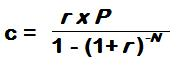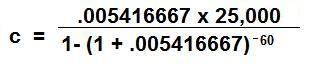CalcuNation

# Car Payment Calculator

## Calculate the monthly loan payment on a vehicle with this Car Payment Calculator.

Principal Loan Amount:
Yearly Interest Rate: %
Total Months of the Loan:
A 5 year loan has 60 months, a 7 year loan has 72 months.

### How do you calculate a car payment?

To calculate the monthly payment on an auto loan use this
car payment formula:c = Monthly Payment

r = Monthly Interest Rate (in Decimal Form) =
(Yearly Interest Rate/100) / 12

P = Principal Amount on the Loan

N = Total # of Months for the loan ( Years on the loan x 12)Example: Monthly payment for 5 year auto loan, with a principal
of \$25,000, and a yearly interest rate of 6.5%:

r = (6.5 / 100) / 12 = .005416667

P = 25,000

N = (5 x 12) = 60

The Monthly Payment is \$489.15

### \$100 Promotion

Win \$100 towards teaching supplies! We want to see your websites and blogs.Enter Here

### Calculator Popups

Scientific Calculator
Simple Calculator

### Calculator Ideas

We use your calculator ideas to create new and useful online calculators.
Submit Calculator Idea